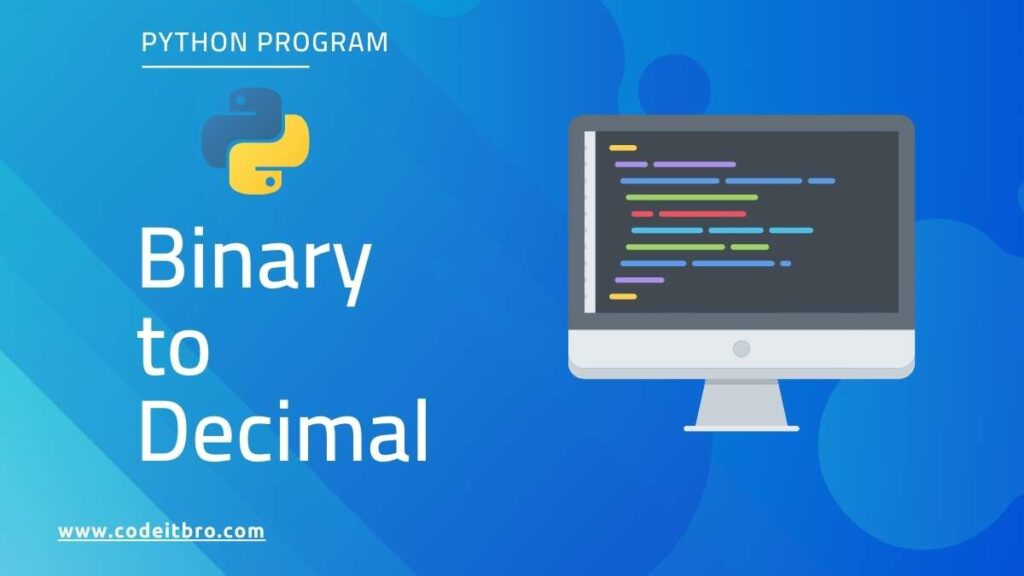# How to Convert Binary to Decimal in Python [5 Methods]

This article will discuss how to convert binary to decimal in Python. So there are different methods to convert binary to decimal in Python. A binary number is represented in the form of 0’s and 1’s, and a decimal number is in the form of an integer value.

## How to Convert Binary to Decimal in PythonLet’s consider a binary number – 1100

```The decimal conversion process is

We have to multiply each value with 2 to raise to the power of values start from 0 to n.

And add all the values   -   (1*2^3)+ (1*2^2)+(0*2^1)+ (0*2^0)

=(1*8)+(1*4)+(0*2)+(0*0)

=8+4

=12```

Hence the decimal value for the binary number 1100 is 12.

## Method 1: Convert Binary to Decimal using int()

int() is used to convert binary numbers to decimals.

Syntax:

`int(binary_number, 2)`

where binary_number is the input binary number.

Steps:

1. Input Binary number
2. Convert binary to a decimal using int()
3. Display the decimal number converted from the binary number

Code:

```#input a number
binary_number= input("Enter an Binary number ")

print("The binary number is ",binary_number)

#convert to decimal
decimal_number= int(binary_number, 2)

print("The decimal number is ",decimal_number)
```

Output:

So we are giving an input – 110110

```Enter an Binary number 110110
The binary number is  110110
The decimal number is  54```

## Method 2: Convert Binary to Decimal without using the inbuilt function

Steps:

1. Input binary number
2. Specify the for loop, and inside that,
3. Divide binary number by 10 to get the remainder
4. Add the remainder to the decimal variable initialized.
5. Divide the binary number by 2 and take only the integer part using the int() function
6. End of the for loop
7. Display the decimal number

Code:

```#get an binary number input from  user
binary_number = int(input("Enter the Binary Number: "))

#create a decimal variable and set to 0
decimal_value=0
#initialize a variable i and set to 1
i = 1

#get the length of the binary number
length = len(str(binary_number))

#logic to convert binary to decimal
for k in range(length):
reminder = binary_number % 10
decimal_value = decimal_value + (reminder * i)
i = i * 2
binary_number = int(binary_number/10)

#display the decimal value
print("Decimal number is  ", decimal_value)
```

Output:

So we are giving an input – 110110

```Enter the Binary Number: 1100
Decimal number is   12```

## Method 3: Convert Binary to Decimal using Recursion

Steps:

1. Input binary number
2. Define the function, and inside that, check the condition and return 0 if the binary number is equal to 0; otherwise, go to step 3.
3. Divide binary number by 10 to get the remainder.
4. Add the remainder to the decimal variable initialized.
5. Divide the binary number by 2 and take only the integer part using the int() function
7. Display the decimal number

Code:

```#Binary_To_Decimal function with 2 parameters
def Binary_To_Decimal(binary_number, exponent=1):
if binary_number== 0:
return 0
else:
#actual conversion lofic
k= binary_number % 10
binary_number= int(binary_number / 10)
k= k * exponent
return k + Binary_To_Decimal(binary_number, exponent * 2)

#input
binary_number = int(input('Enter a binary number: '))

#output
print('The decimal value is ', Binary_To_Decimal(binary_number))
```

Output:

So we are giving an input – 1100

```Enter a binary number: 1100
The decimal value is  12
```

## Method 4: Convert Binary List to Decimal

In this case, we are going to convert some binary numbers to decimal through a  list in Python

int() is used to convert binary numbers to decimals.

Syntax:

` (int(str(i), 2) for i in binary_numbers)`

where binary_number is the input binary number.

Steps:

1. Input Binary numbers from a list
2. Iterate the list of binary numbers and convert it to a decimal using the int() function. Place str() to convert the binary values from integer to string
3. Use for loop to get the decimal values from binary values

Code:

```#binary_numbers
binary_numbers = [11,00,1110,111000,10001,110]

#convert to decimal
decimal_data= (int(str(i), 2) for i in binary_numbers)

#display
for i in decimal_data:
print(i)
```

Output:

So we are giving 6 binary input values.

```3
0
14
56
17
6
```

## Method 5: Convert Binary string List to Decimal

In this method, we are going to convert some binary numbers of string type  to decimal through a  list in Python

int() is used to convert binary numbers to decimal.

Syntax:

`(int(i, 2) for i in binary_numbers)`

where binary_number is the input binary number.

Steps:

1. Input Binary numbers from a list
2. Iterate the list of binary numbers and convert them to decimals using the int() function.
3. Use for loop to get the decimal values from binary values

Code:

```#binary_numbers
binary_numbers = ['11','00','1110','111000','10001','110']

#convert to decimal
decimal_data= (int(i, 2) for i in binary_numbers)

#display
for i in decimal_data:
print(i)
```

Output:

So we are giving 6 binary input values.

```3
0
14
56
17
6
```

So, these are the ways to convert Binary values to Decimal values in Python.